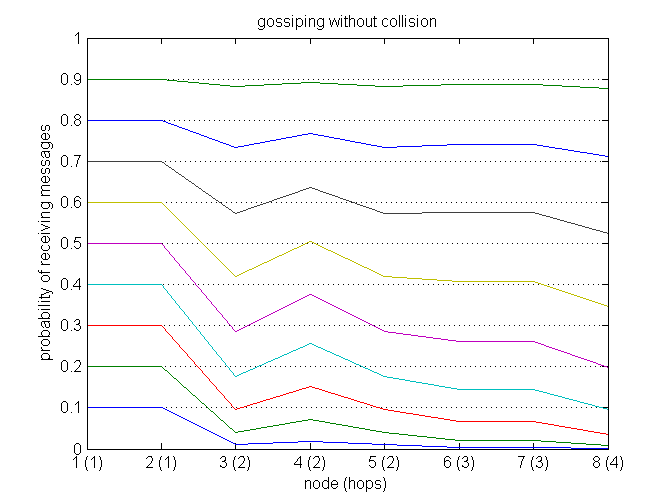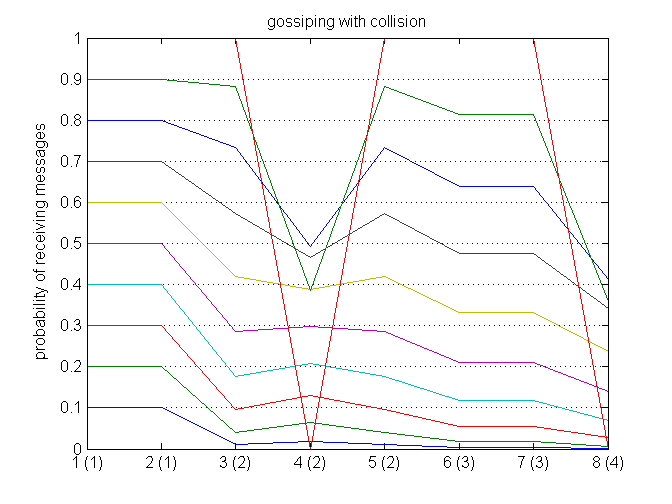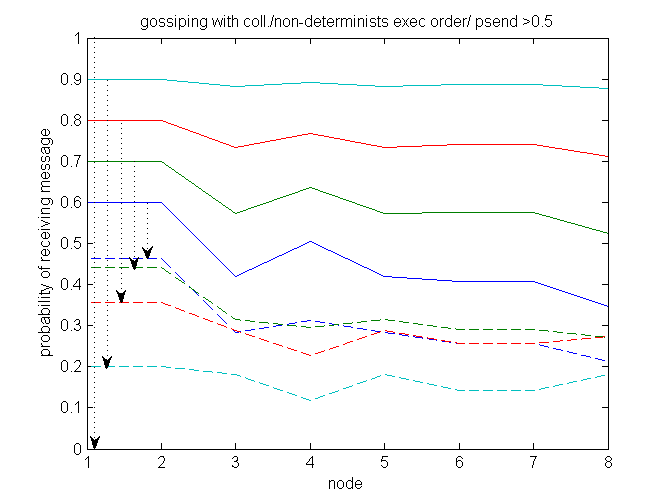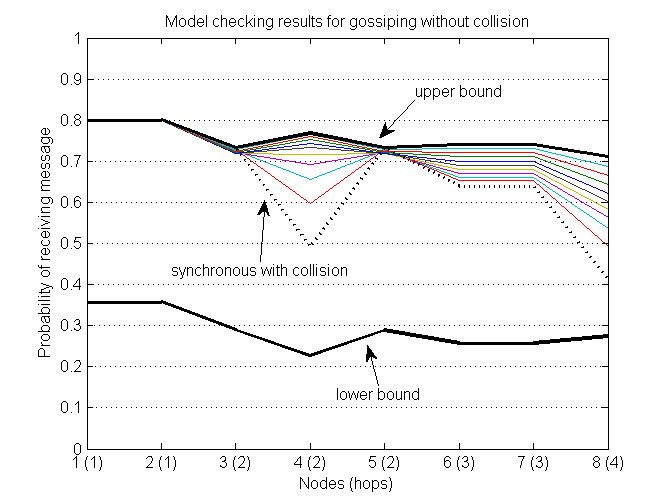[Contributed by Ansgar Fehnker and Peng Gao]

Related publications: [FG06]

### Introduction

This work describes formal probabilistic models of flooding and gossiping protocols, and explores the influence of different modelling choices and assumptions on the results of performance analysis. We use PRISM, for the formal analysis of protocols and small network topologies, and used in addition Monte-Carlo simulation, implemented in Matlab, to establish if the results and effects found during formal analysis extend to larger networks.

Many protocols for wireless sensor networks employ some form of the very simple flooding protocol to acquire or distribute information. Flooding a message means that each node that receives a message, propagates it to all its neighbours by broadcast. This introduces an unnecessary redundancy, because a node may receive a message multiple times. Gossiping protocols introduce a random element to reduce this redundancy. Gossiping means that each node decides with a certain probability to forward a message on or not. This reduces the probability for node to receive a message multiple times, thus the redundancy and cost.

We different modelling choices and its influence on the results, in particular the effect of collisions, unreliable channels versus probabilistic broadcast, and the influence of timing. This analysis can explain why the performance result of gossiping for a perfectly synchronised network without collision, are similar to the result on a network with randomised delay and collision, although the actual behaviour is vastly different.

The following are the models and a brief description of the models used in [FG06].

### Basic Protocol

Gossiping is a simple protocol that uses probabilistic broadcast to send or request information in a wireless network. The gossiping protocol can be informally summarised as follows:

• The source node broadcasts the message or request to all its neighbours. It then proceeds to sleep.
• Nodes that receive a message choose
• with probability psend to forward the received message, and
• with probability 1 - psend to ignore the message.
In either case the node proceeds to sleep.

Gossiping is equal to flooding, if psend is equal to 1.

### Synchronous without collision

The baseline model is a synchronous PRISM model for gossiping without collision. Synchronous means that sending and receiving is organized in rounds. All nodes that receive a message at one point in time, will choose at the same time whether to forward the message, and if the decide to forward it, they all forward it at the same time.

The following models are for a 3 by 3 grid, and only direct neighbours can receive each others messages.

One node, node0 is the gateway and sends regardless of whether it received a message. All other nodes run the plain gossiping model.

```dtmc

const double psend =0.8;

module node0
active0:[0..1] init 1;
send0:  [0..1] init 0;

[tick] active0=1 & send0=0 -> psend:(active0'=1)&(send0'=1)+(1-psend):(active0'=0)&(send0'=0);
[tick] active0=1 & send0=1 -> (send0'=0)& (active0'=0);
[tick] active0=0  -> (send0'=0)& (active0'=0);
endmodule

module node1
active1:[0..1] init 1;
send1:  [0..1] init 0;

[tick] active1=1 & send1=0 & send0+send2+send4 >=1 -> psend:(active1'=1)&(send1'=1)+(1-psend):(active1'=0)&(send1'=0);
[tick] active1=1 & send1=0 & send0+send2+send4 <1 -> (active1'=1)& (send1'=0);
[tick] active1=1 & send1=1 -> (send1'=0)& (active1'=0);
[tick] active1=0 -> (send1'=0)& (active1'=0);
endmodule

module node2
active2:[0..1] init 1;
send2:  [0..1] init 0;

[tick] active2=1 & send2=0 & send1+send5 >= 1 -> psend:(active2'=1)&(send2'=1)+(1-psend):(active2'=0)&(send2'=0);
[tick] active2=1 & send2=0 & send1+send5 < 1 -> (active2'=1)& (send2'=0);
[tick] active2=1 & send2=1   -> (send2'=0)& (active2'=0);
[tick] active2=0  -> (send2'=0)& (active2'=0);
endmodule

module node3
active3:[0..1] init 1;
send3:  [0..1] init 0;

[tick] active3=1 & send3=0 & send0+send4+ send6 >=1 -> psend:(active3'=1)&(send3'=1)+(1-psend):(active3'=0)&(send3'=0);
[tick] active3=1 & send3=0 & send0+send4+ send6 <1 -> (active3'=1)& (send3'=0);
[tick] active3=1 & send3=1    -> (send3'=0)& (active3'=0);
[tick] active3=0  -> (send3'=0)& (active3'=0);
endmodule

module node4
active4:[0..1] init 1;
send4:  [0..1] init 0;

[tick] active4=1 & send4=0 & send1+send3+ send5 +send7 >=1 -> psend:(active4'=1)&(send4'=1)+(1-psend):(active4'=0)&(send4'=0);
[tick] active4=1 & send4=0 & send1+send3+ send5 +send7 <1 -> (active4'=1) & (send4'=0);
[tick] active4=1 & send4=1 -> (send4'=0)& (active4'=0);
[tick] active4=0  -> (send4'=0)& (active4'=0);
endmodule

module node5
active5:[0..1] init 1;
send5:  [0..1] init 0;

[tick] active5=1 & send5=0 & send2+send4+ send8 >=1 -> psend:(active5'=1)&(send5'=1)+(1-psend):(active5'=0)&(send5'=0);
[tick] active5=1 & send5=0 & send2+send4+ send8 < 1 -> (active5'=1) & (send5'=0);
[tick] active5=1 & send5=1 -> (send5'=0)& (active5'=0);
[tick] active5=0  -> (send5'=0)& (active5'=0);
endmodule

module node6
active6:[0..1] init 1;
send6:  [0..1] init 0;

[tick] active6=1 & send6=0 & send3+send7 >=1 -> psend:(active6'=1)&(send6'=1)+(1-psend):(active6'=0)&(send6'=0);
[tick] active6=1 & send6=0 & send3+send7 < 1 -> (active6'=1) & (send6'=0);
[tick] active6=1 & send6=1    -> (send6'=0)& (active6'=0);
[tick] active6=0  -> (send6'=0)& (active6'=0);
endmodule

module node7
active7:[0..1] init 1;
send7:  [0..1] init 0;

[tick] active7=1 & send7=0 & send4+send6+ send8 >=1 -> psend:(active7'=1)&(send7'=1)+(1-psend):(active7'=0)&(send7'=0);
[tick] active7=1 & send7=0 & send4+send6+ send8 < 1 -> (active7'=1) & (send7'=0);
[tick] active7=1 & send7=1    -> (send7'=0)& (active7'=0);
[tick] active7=0  -> (send7'=0)& (active7'=0);
endmodule

module node8
active8:[0..1] init 1;
send8:  [0..1] init 0;

[tick] active8=1 & send8=0 & send5+send7 >=1 -> psend:(active8'=1)&(send8'=1)+(1-psend):(active8'=0)&(send8'=0);
[tick] active8=1 & send8=0 & send5+send7 < 1 -> (active8'=1) & (send8'=0);
[tick] active8=1 & send8=1    -> (send8'=0)& (active8'=0);
[tick] active8=0  -> (send8'=0)& (active8'=0);
endmodule
```

### Synchronous with collision

The first model assumes that a node receives a message when at least one of the neighbours sends. However, in wireless networks, if two or more neighbours send, all a node will receive is noise. The guard of the node changes from send0+send2+send4 >= 1 to send0+send2+send4 = 1

```dtmc

const double psend;

module node0
active0:[0..1] init 1;
send0:  [0..1] init 0;

[tick] active0=1 & send0=0 -> psend:(active0'=1)&(send0'=1)+(1-psend):(active0'=0)&(send0'=0);
[tick] active0=1 & send0=1 -> (send0'=0)& (active0'=0);
[tick] active0=0  -> (send0'=0)& (active0'=0);
endmodule

module node1
active1:[0..1] init 1;
send1:  [0..1] init 0;

[tick] active1=1 & send1=0 & send0+send2+send4 = 1 -> psend:(active1'=1)&(send1'=1)+(1-psend):(active1'=0)&(send1'=0);
[tick] active1=1 & send1=0 & send0+send2+send4 !=1 -> (active1'=1)& (send1'=0);
[tick] active1=1 & send1=1 -> (send1'=0)& (active1'=0);
[tick] active1=0 -> (send1'=0)& (active1'=0);
endmodule

module node2
active2:[0..1] init 1;
send2:  [0..1] init 0;

[tick] active2=1 & send2=0 & send1+send5 = 1 -> psend:(active2'=1)&(send2'=1)+(1-psend):(active2'=0)&(send2'=0);
[tick] active2=1 & send2=0 & send1+send5 !=1 -> (active2'=1)& (send2'=0);
[tick] active2=1 & send2=1    -> (send2'=0)& (active2'=0);
[tick] active2=0  -> (send2'=0)& (active2'=0);
endmodule

module node3
active3:[0..1] init 1;
send3:  [0..1] init 0;

[tick] active3=1 & send3=0 & send0+send4+ send6 = 1 -> psend:(active3'=1)&(send3'=1)+(1-psend):(active3'=0)&(send3'=0);
[tick] active3=1 & send3=0 & send0+send4+ send6 !=1 -> (active3'=1)& (send3'=0);
[tick] active3=1 & send3=1    -> (send3'=0)& (active3'=0);
[tick] active3=0  -> (send3'=0)& (active3'=0);
endmodule

module node4
active4:[0..1] init 1;
send4:  [0..1] init 0;

[tick] active4=1 & send4=0 & send1+send3+ send5 +send7 = 1 -> psend:(active4'=1)&(send4'=1)+(1-psend):(active4'=0)&(send4'=0);
[tick] active4=1 & send4=0 & send1+send3+ send5 +send7 !=1 -> (active4'=1) & (send4'=0);
[tick] active4=1 & send4=1 -> (send4'=0)& (active4'=0);
[tick] active4=0  -> (send4'=0)& (active4'=0);
endmodule

module node5
active5:[0..1] init 1;
send5:  [0..1] init 0;

[tick] active5=1 & send5=0 & send2+send4+ send8 = 1 -> psend:(active5'=1)&(send5'=1)+(1-psend):(active5'=0)&(send5'=0);
[tick] active5=1 & send5=0 & send2+send4+ send8 !=1 -> (active5'=1) & (send5'=0);
[tick] active5=1 & send5=1 -> (send5'=0)& (active5'=0);
[tick] active5=0  -> (send5'=0)& (active5'=0);
endmodule

module node6
active6:[0..1] init 1;
send6:  [0..1] init 0;

[tick] active6=1 & send6=0 & send3+send7 = 1 -> psend:(active6'=1)&(send6'=1)+(1-psend):(active6'=0)&(send6'=0);
[tick] active6=1 & send6=0 & send3+send7 !=1 -> (active6'=1) & (send6'=0);
[tick] active6=1 & send6=1    -> (send6'=0)& (active6'=0);
[tick] active6=0  -> (send6'=0)& (active6'=0);
endmodule

module node7
active7:[0..1] init 1;
send7:  [0..1] init 0;

[tick] active7=1 & send7=0 & send4+send6+ send8 = 1 -> psend:(active7'=1)&(send7'=1)+(1-psend):(active7'=0)&(send7'=0);
[tick] active7=1 & send7=0 & send4+send6+ send8 !=1 -> (active7'=1) & (send7'=0);
[tick] active7=1 & send7=1    -> (send7'=0)& (active7'=0);
[tick] active7=0  -> (send7'=0)& (active7'=0);
endmodule

module node8
active8:[0..1] init 1;
send8:  [0..1] init 0;

[tick] active8=1 & send8=0 & send5+send7 = 1 -> psend:(active8'=1)&(send8'=1)+(1-psend):(active8'=0)&(send8'=0);
[tick] active8=1 & send8=0 & send5+send7 !=1 -> (active8'=1) & (send8'=0);
[tick] active8=1 & send8=1    -> (send8'=0)& (active8'=0);
[tick] active8=0  -> (send8'=0)& (active8'=0);
endmodule
```

### Lossy Channel

In the first two models a node either send to all of its neighbours or to none. In a lossy channel model each link between two nodes has its own channel, and messages can get along each of the channel, i.e. reception of a message by different nodes is no longer correlated. To model this we introduce a variable 'buf' for each channel, that contains the value 1, if a message has been sent. The model includes one module for the channel between each pair of neighboring nodes and a scheduler is used to alternate between updates of the nodes and updates of the channels.

```dtmc

const double psend =1;
const double precv ;

module scheduler
turn: bool init true;

[tick] !turn ->(turn' = true);
[tock] turn  -> (turn'= false);
endmodule

// first horizontal
module chan01
buff01: [0..1] init 0;

[tock] send0=1 | send1= 1 -> precv:(buff01'=1)+ (1-precv):(buff01'=0);
[tock] send0!=1 & send1!=1 -> (buff01'=0);
endmodule

module chan12
buff12: [0..1] init 0;

[tock] send1=1 | send2= 1 -> precv:(buff12'=1)+ (1-precv):(buff12'=0);
[tock] send1!=1 & send2!=1 -> (buff12'=0);
endmodule

// first vertical
module chan03
buff03: [0..1] init 0;

[tock] send0=1 | send3= 1 -> precv:(buff03'=1)+ (1-precv):(buff03'=0);
[tock] send0!=1 & send3!=1 -> (buff03'=0);
endmodule

module chan14
buff14: [0..1] init 0;

[tock] send1=1 | send4= 1 -> precv:(buff14'=1)+ (1-precv):(buff14'=0);
[tock] send1!=1 & send4!=1 -> (buff14'=0);
endmodule

module chan25
buff25: [0..1] init 0;

[tock] send2=1 | send5= 1 -> precv:(buff25'=1)+ (1-precv):(buff25'=0);
[tock] send2!=1 & send5!=1 -> (buff25'=0);
endmodule

// second horizontal
module chan34
buff34: [0..1] init 0;

[tock] send3=1 | send4= 1 -> precv:(buff34'=1)+ (1-precv):(buff34'=0);
[tock] send3!=1 & send4!=1 -> (buff34'=0);
endmodule

module chan45
buff45: [0..1] init 0;

[tock] send4=1 | send5= 1 -> precv:(buff45'=1)+ (1-precv):(buff45'=0);
[tock] send4!=1 & send5!=1 -> (buff45'=0);
endmodule

// second vertical
module chan36
buff36: [0..1] init 0;

[tock] send3=1 | send6= 1 -> precv:(buff36'=1)+ (1-precv):(buff36'=0);
[tock] send3!=1 & send6!=1 -> (buff36'=0);
endmodule

module chan47
buff47: [0..1] init 0;

[tock] send4=1 | send7= 1 -> precv:(buff47'=1)+ (1-precv):(buff47'=0);
[tock] send4!=1 & send7!=1 -> (buff47'=0);
endmodule

module chan58
buff58: [0..1] init 0;

[tock] send5=1 | send8= 1 -> precv:(buff58'=1)+ (1-precv):(buff58'=0);
[tock] send5!=1 & send8!=1 -> (buff58'=0);
endmodule

// second horizontal
module chan67
buff67: [0..1] init 0;

[tock] send6=1 | send7= 1 -> precv:(buff67'=1)+ (1-precv):(buff67'=0);
[tock] send6!=1 & send7!=1 -> (buff67'=0);
endmodule

module chan78
buff78: [0..1] init 0;

[tock] send7=1 | send8= 1 -> precv:(buff78'=1)+ (1-precv):(buff78'=0);
[tock] send7!=1 & send8!=1 -> (buff78'=0);
endmodule

// nodes

module node0
active0:[0..1] init 1;
send0:  [0..1] init 0;

[tick] active0=1 & send0=0 -> psend:(active0'=1)&(send0'=1)+(1-psend):(active0'=0)&(send0'=0);
[tick] active0=1 & send0=1 -> (send0'=0)& (active0'=0);
[tick] active0=0  -> (send0'=0)& (active0'=0);
endmodule

module node1
active1:[0..1] init 1;
send1:  [0..1] init 0;

[tick] active1=1 & send1=0 &  1=(buff01 +buff12 +buff14)-> psend:(active1'=1)&(send1'=1)+(1-psend):(active1'=0)&(send1'=0);
[tick] active1=1 & send1=0 &  1!=(buff01 +buff12 +buff14) -> (active1'=1)& (send1'=0);
[tick] active1=1 & send1=1 -> (send1'=0)& (active1'=0);
[tick] active1=0 -> (send1'=0)& (active1'=0);
endmodule

module node2
active2:[0..1] init 1;
send2:  [0..1] init 0;

[tick] active2=1 & send2=0 &  1=(buff12 +buff25) -> psend:(active2'=1)&(send2'=1)+(1-psend):(active2'=0)&(send2'=0);
[tick] active2=1 & send2=0 & 1!=(buff12 +buff25) -> (active2'=1)& (send2'=0);
[tick] active2=1 & send2=1    -> (send2'=0)& (active2'=0);
[tick] active2=0  -> (send2'=0)& (active2'=0);
endmodule

module node3
active3:[0..1] init 1;
send3:  [0..1] init 0;

[tick] active3=1 & send3=0 &  1=(buff03 +buff34 +buff36) -> psend:(active3'=1)&(send3'=1)+(1-psend):(active3'=0)&(send3'=0);
[tick] active3=1 & send3=0 & 1!=(buff03 +buff34 +buff36) -> (active3'=1)& (send3'=0);
[tick] active3=1 & send3=1    -> (send3'=0)& (active3'=0);
[tick] active3=0  -> (send3'=0)& (active3'=0);
endmodule

module node4
active4:[0..1] init 1;
send4:  [0..1] init 0;

[tick] active4=1 & send4=0 &  1=(buff14 +buff34 +buff45 +buff47) -> psend:(active4'=1)&(send4'=1)+(1-psend):(active4'=0)&(send4'=0);
[tick] active4=1 & send4=0 & 1!=(buff14 +buff34 +buff45 +buff47) -> (active4'=1) & (send4'=0);
[tick] active4=1 & send4=1 -> (send4'=0)& (active4'=0);
[tick] active4=0  -> (send4'=0)& (active4'=0);
endmodule

module node5
active5:[0..1] init 1;
send5:  [0..1] init 0;

[tick] active5=1 & send5=0 &  1=(buff25 +buff45 +buff58) -> psend:(active5'=1)&(send5'=1)+(1-psend):(active5'=0)&(send5'=0);
[tick] active5=1 & send5=0 & 1!=(buff25 +buff45 +buff58) -> (active5'=1) & (send5'=0);
[tick] active5=1 & send5=1 -> (send5'=0)& (active5'=0);
[tick] active5=0  -> (send5'=0)& (active5'=0);
endmodule

module node6
active6:[0..1] init 1;
send6:  [0..1] init 0;

[tick] active6=1 & send6=0 & 1=(buff36 +buff67) -> psend:(active6'=1)&(send6'=1)+(1-psend):(active6'=0)&(send6'=0);
[tick] active6=1 & send6=0 & 1!=(buff36 +buff67) -> (active6'=1) & (send6'=0);
[tick] active6=1 & send6=1    -> (send6'=0)& (active6'=0);
[tick] active6=0  -> (send6'=0)& (active6'=0);
endmodule

module node7
active7:[0..1] init 1;
send7:  [0..1] init 0;

[tick] active7=1 & send7=0 &  1=(buff67 +buff47 +buff78) -> psend:(active7'=1)&(send7'=1)+(1-psend):(active7'=0)&(send7'=0);
[tick] active7=1 & send7=0 & 1!=(buff67 +buff47 +buff78) -> (active7'=1) & (send7'=0);
[tick] active7=1 & send7=1    -> (send7'=0)& (active7'=0);
[tick] active7=0  -> (send7'=0)& (active7'=0);
endmodule

module node8
active8:[0..1] init 1;
send8:  [0..1] init 0;

[tick] active8=1 & send8=0 &  1=(buff58 +buff78) -> psend:(active8'=1)&(send8'=1)+(1-psend):(active8'=0)&(send8'=0);
[tick] active8=1 & send8=0 & 1!=(buff58 +buff78) -> (active8'=1) & (send8'=0);
[tick] active8=1 & send8=1    -> (send8'=0)& (active8'=0);
[tick] active8=0  -> (send8'=0)& (active8'=0);
endmodule
```

### Asynchronous execution

All models presented thus far have been synchronous in the sense that all nodes (and all channels) update their state synchronously. Nodes that receive a message at the same time, will respond to it at the same time. In a wireless network such synchronicity is typically absent. Nodes operate independently and have each their own clock that might drift or jitter.

The integer variable send1 now records if no message, one message, or more than one message has been received, with send1=2 if a collision occurred. If a node detects a collision, it may return to the initial state. Since this is an internal action, it has no synchronisation label. If a node did receive exactly one message it can broadcast. Node 1 synchronises on label msg1 with the neighbouring nodes to realise a broadcast.

```mdp

const double psend;

module node0
active0:[0..1] init 1;
send0:  [0..1] init 0;

[] active0=1 & send0=0 -> psend:(active0'=1)&(send0'=1)+(1-psend):(active0'=0)&(send0'=0);
[msg0] active0=1 & send0=1 -> (send0'=0)& (active0'=0);
[msg1] active0=0 -> (send0'=0)& (active0'=0);
[msg3] active0=0 -> (send0'=0)& (active0'=0);
endmodule

module node1
active1:[0..1] init 1;
send1:  [0..2] init 0;

[msg0] active1=1 & send1=0 -> psend:(active1'=1)&(send1'=1)+(1-psend):(active1'=0)&(send1'=0);
[msg2] active1=1 & send1=0 -> psend:(active1'=1)&(send1'=1)+(1-psend):(active1'=0)&(send1'=0);
[msg4] active1=1 & send1=0 -> psend:(active1'=1)&(send1'=1)+(1-psend):(active1'=0)&(send1'=0);
[msg0] active1=1 & send1!=0  -> (active1'=1) & (send1'=2);
[msg2] active1=1 & send1!=0  -> (active1'=1) & (send1'=2);
[msg4] active1=1 & send1!=0  -> (active1'=1) & (send1'=2);
[]     active1=1 & send1 =2  -> (active1'=1) & (send1'=0);
[msg1] active1=1 & send1=1 -> (send1'=0)& (active1'=0);
[msg0] active1=0 -> (send1'=0)& (active1'=0);
[msg2] active1=0 -> (send1'=0)& (active1'=0);
[msg4] active1=0 -> (send1'=0)& (active1'=0);
endmodule

module node2
active2:[0..1] init 1;
send2:  [0..2] init 0;

[msg1] active2=1 & send2=0  -> psend:(active2'=1)&(send2'=1)+(1-psend):(active2'=0)&(send2'=0);
[msg5] active2=1 & send2=0  -> psend:(active2'=1)&(send2'=1)+(1-psend):(active2'=0)&(send2'=0);
[msg1] active2=1 & send2!=0  -> (active2'=1) & (send2'=2);
[msg5] active2=1 & send2!=0  -> (active2'=1) & (send2'=2);
[]     active2=1 & send2 =2  -> (active2'=1) & (send2'=0);
[msg2] active2=1 & send2=1    -> (send2'=0)& (active2'=0);
[msg1] active2=0 -> (send2'=0)& (active2'=0);
[msg5] active2=0 -> (send2'=0)& (active2'=0);
endmodule

module node3
active3:[0..1] init 1;
send3:  [0..2] init 0;

[msg0] active3=1 & send3=0    -> psend:(active3'=1)&(send3'=1)+(1-psend):(active3'=0)&(send3'=0);
[msg4] active3=1 & send3=0    -> psend:(active3'=1)&(send3'=1)+(1-psend):(active3'=0)&(send3'=0);
[msg6] active3=1 & send3=0    -> psend:(active3'=1)&(send3'=1)+(1-psend):(active3'=0)&(send3'=0);
[msg0] active3=1 & send3!=0  -> (active3'=1) & (send3'=2);
[msg4] active3=1 & send3!=0  -> (active3'=1) & (send3'=2);
[msg6] active3=1 & send3!=0  -> (active3'=1) & (send3'=2);
[]     active3=1 & send3 =2  -> (active3'=1) & (send3'=0);
[msg3] active3=1 & send3=1    -> (send3'=0)& (active3'=0);
[msg0] active3=0 -> (send3'=0)& (active3'=0);
[msg4] active3=0 -> (send3'=0)& (active3'=0);
[msg6] active3=0 -> (send3'=0)& (active3'=0);
endmodule

module node4
active4:[0..1] init 1;
send4:  [0..2] init 0;

[msg1] active4=1 & send4=0 -> psend:(active4'=1)&(send4'=1)+(1-psend):(active4'=0)&(send4'=0);
[msg3] active4=1 & send4=0 -> psend:(active4'=1)&(send4'=1)+(1-psend):(active4'=0)&(send4'=0);
[msg5] active4=1 & send4=0 -> psend:(active4'=1)&(send4'=1)+(1-psend):(active4'=0)&(send4'=0);
[msg7] active4=1 & send4=0 -> psend:(active4'=1)&(send4'=1)+(1-psend):(active4'=0)&(send4'=0);
[msg1] active4=1 & send4!=0  -> (active4'=1) & (send4'=2);
[msg3] active4=1 & send4!=0  -> (active4'=1) & (send4'=2);
[msg5] active4=1 & send4!=0  -> (active4'=1) & (send4'=2);
[msg7] active4=1 & send4!=0  -> (active4'=1) & (send4'=2);
[]     active4=1 & send4 =2  -> (active4'=1) & (send4'=0);
[msg4] active4=1 & send4=1 -> (send4'=0)& (active4'=0);
[msg1] active4=0 -> (send4'=0)& (active4'=0);
[msg3] active4=0 -> (send4'=0)& (active4'=0);
[msg5] active4=0 -> (send4'=0)& (active4'=0);
[msg7] active4=0 -> (send4'=0)& (active4'=0);
endmodule

module node5
active5:[0..1] init 1;
send5:  [0..2] init 0;

[msg2] active5=1 & send5=0 -> psend:(active5'=1)&(send5'=1)+(1-psend):(active5'=0)&(send5'=0);
[msg4] active5=1 & send5=0 -> psend:(active5'=1)&(send5'=1)+(1-psend):(active5'=0)&(send5'=0);
[msg8] active5=1 & send5=0 -> psend:(active5'=1)&(send5'=1)+(1-psend):(active5'=0)&(send5'=0);
[msg2] active5=1 & send5!=0  -> (active5'=1) & (send5'=2);
[msg4] active5=1 & send5!=0  -> (active5'=1) & (send5'=2);
[msg8] active5=1 & send5!=0  -> (active5'=1) & (send5'=2);
[]     active5=1 & send5 =2  -> (active5'=1) & (send5'=0);
[msg5] active5=1 & send5=1 -> (send5'=0)& (active5'=0);
[msg2] active5=0 -> (send5'=0)& (active5'=0);
[msg4] active5=0 -> (send5'=0)& (active5'=0);
[msg8] active5=0 -> (send5'=0)& (active5'=0);
endmodule

module node6
active6:[0..1] init 1;
send6:  [0..2] init 0;

[msg3] active6=1 & send6=0 -> psend:(active6'=1)&(send6'=1)+(1-psend):(active6'=0)&(send6'=0);
[msg7] active6=1 & send6=0 -> psend:(active6'=1)&(send6'=1)+(1-psend):(active6'=0)&(send6'=0);
[msg3] active6=1 & send6!=0  -> (active6'=1) & (send6'=2);
[msg7] active6=1 & send6!=0  -> (active6'=1) & (send6'=2);
[]     active6=1 & send6 =2  -> (active6'=1) & (send6'=0);
[msg6] active6=1 & send6=1 -> (send6'=0)& (active6'=0);
[msg3] active6=0 -> (send6'=0)& (active6'=0);
[msg7] active6=0 -> (send6'=0)& (active6'=0);
endmodule

module node7
active7:[0..1] init 1;
send7:  [0..2] init 0;

[msg4] active7=1 & send7=0 -> psend:(active7'=1)&(send7'=1)+(1-psend):(active7'=0)&(send7'=0);
[msg6] active7=1 & send7=0 -> psend:(active7'=1)&(send7'=1)+(1-psend):(active7'=0)&(send7'=0);
[msg8] active7=1 & send7=0 -> psend:(active7'=1)&(send7'=1)+(1-psend):(active7'=0)&(send7'=0);
[msg4] active7=1 & send7!=0  -> (active7'=1) & (send7'=2);
[msg6] active7=1 & send7!=0  -> (active7'=1) & (send7'=2);
[msg8] active7=1 & send7!=0  -> (active7'=1) & (send7'=2);
[]     active7=1 & send7 =2  -> (active7'=1) & (send7'=0);
[msg7] active7=1 & send7=1 -> (send7'=0)& (active7'=0);
[msg4] active7=0 -> (send7'=0)& (active7'=0);
[msg6] active7=0 -> (send7'=0)& (active7'=0);
[msg8] active7=0 -> (send7'=0)& (active7'=0);
endmodule

module node8
active8:[0..1] init 1;
send8:  [0..2] init 0;

[msg5] active8=1 & send8=0 -> psend:(active8'=1)&(send8'=1)+(1-psend):(active8'=0)&(send8'=0);
[msg7] active8=1 & send8=0 -> psend:(active8'=1)&(send8'=1)+(1-psend):(active8'=0)&(send8'=0);
[msg5] active8=1 & send8!=0  -> (active8'=1) & (send8'=2);
[msg7] active8=1 & send8!=0  -> (active8'=1) & (send8'=2);
[]     active8=1 & send8 =2  -> (active8'=1) & (send8'=0);
[msg8] active8=1 & send8=1 -> (send8'=0)& (active8'=0);
[msg5] active8=0 -> (send8'=0)& (active8'=0);
[msg7] active8=0 -> (send8'=0)& (active8'=0);
endmodule
```

### Unreliable Timing

Assuming any execution order is a very conservative assumption. Although clocks may drift and jitter, they all proceed positive rate in about the same range. In this section we will consider different timing models for gossiping that contain different types of random delay.

To model delay we use a simple memoryless delay. This means that the when a message receives a message it can decide to delay transmission with a certain probability pdelay.

```dtmc

const double psend =0.8;
const double pdelay =0.5;

module node0
active0:[0..1] init 1;
send0:  [0..1] init 0;

[tick] active0=1 & send0=0 -> psend:(active0'=1)&(send0'=1)+(1-psend):(active0'=0)&(send0'=0);
[tick] active0=1 & send0=1 -> (send0'=0)& (active0'=0);
[tick] active0=0  -> (send0'=0)& (active0'=0);
endmodule

module node1
active1:[0..2] init 1;
send1:  [0..1] init 0;

[tick] active1=1 & send1=0 & send0+send2+send4 =1 -> (1-pdelay)* psend: (active1'=1)&(send1'=1)
+(1-pdelay)*(1-psend):(active1'=0)&(send1'=0)
+   pdelay:           (active1'=2)&(send1'=0);
[tick] active1=2                                   -> (1-pdelay)*psend: (active1'=1)&(send1'=1)
+(1-pdelay)*(1-psend):(active1'=0)&(send1'=0)
+   pdelay:           (active1'=2)&(send1'=0);
[tick] active1=1 & send1=0 &  send0+send2+send4 !=1 -> (active1'=1)& (send1'=0);
[tick] active1=1 & send1=1 -> (send1'=0)& (active1'=0);
[tick] active1=0           -> (send1'=0)& (active1'=0);
endmodule

module node2
active2:[0..2] init 1;
send2:  [0..1] init 0;

[tick] active2=1 & send2=0 & send1+send5 =1 ->       (1-pdelay)*psend: (active2'=1)&(send2'=1)
+(1-pdelay)*(1-psend):(active2'=0)&(send2'=0)
+   pdelay:           (active2'=2)&(send2'=0);
[tick] active2=2                                  ->  (1-pdelay)*psend: (active2'=1)&(send2'=1)
+(1-pdelay)*(1-psend):(active2'=0)&(send2'=0)
+   pdelay:           (active2'=2)&(send2'=0);
[tick] active2=1 & send2=0 & send1+send5 !=1 -> (active2'=1)& (send2'=0);
[tick] active2=1 & send2=1   -> (send2'=0)& (active2'=0);
[tick] active2=0  -> (send2'=0)& (active2'=0);
endmodule

module node3
active3:[0..2] init 1;
send3:  [0..1] init 0;

[tick] active3=1 & send3=0 & send0+send4+ send6  =1 ->       (1-pdelay)*psend: (active3'=1)&(send3'=1)
+(1-pdelay)*(1-psend):(active3'=0)&(send3'=0)
+   pdelay:           (active3'=2)&(send3'=0);
[tick] active3=2                                   ->       (1-pdelay)*psend: (active3'=1)&(send3'=1)
+(1-pdelay)*(1-psend):(active3'=0)&(send3'=0)
+   pdelay:           (active3'=2)&(send3'=0);
[tick] active3=1 & send3=0 & send0+send4+ send6 !=1 -> (active3'=1)& (send3'=0);
[tick] active3=1 & send3=1    -> (send3'=0)& (active3'=0);
[tick] active3=0  -> (send3'=0)& (active3'=0);
endmodule

module node4
active4:[0..2] init 1;
send4:  [0..1] init 0;

[tick] active4=1 & send4=0 & send1+send3+send5+send7 =1 ->       (1-pdelay)*psend: (active4'=1)&(send4'=1)
+(1-pdelay)*(1-psend):(active4'=0)&(send4'=0)
+   pdelay:           (active4'=2)&(send4'=0);
[tick] active4=2                                   ->       (1-pdelay)*psend: (active4'=1)&(send4'=1)
+(1-pdelay)*(1-psend):(active4'=0)&(send4'=0)
+   pdelay:           (active4'=2)&(send4'=0);
[tick] active4=1 & send4=0 & send1+send3+ send5 +send7 !=1 -> (active4'=1) & (send4'=0);
[tick] active4=1 & send4=1 -> (send4'=0)& (active4'=0);
[tick] active4=0  -> (send4'=0)& (active4'=0);
endmodule

module node5
active5:[0..2] init 1;
send5:  [0..1] init 0;

[tick] active5=1 & send5=0 & send2+send4+ send8=1 ->       (1-pdelay)*psend: (active5'=1)&(send5'=1)
+(1-pdelay)*(1-psend):(active5'=0)&(send5'=0)
+   pdelay:           (active5'=2)&(send5'=0);
[tick] active5=2                                   ->       (1-pdelay)*psend: (active5'=1)&(send5'=1)
+(1-pdelay)*(1-psend):(active5'=0)&(send5'=0)
+   pdelay:           (active5'=2)&(send5'=0);
[tick] active5=1 & send5=0 & send2+send4+ send8 !=1 -> (active5'=1) & (send5'=0);
[tick] active5=1 & send5=1 -> (send5'=0)& (active5'=0);
[tick] active5=0  -> (send5'=0)& (active5'=0);
endmodule

module node6
active6:[0..2] init 1;
send6:  [0..1] init 0;

[tick] active6=1 & send6=0 & send3+send7=1  ->       (1-pdelay)*psend: (active6'=1)&(send6'=1)
+(1-pdelay)*(1-psend):(active6'=0)&(send6'=0)
+   pdelay:           (active6'=2)&(send6'=0);
[tick] active6=2                                   ->       (1-pdelay)*psend: (active6'=1)&(send6'=1)
+(1-pdelay)*(1-psend):(active6'=0)&(send6'=0)
+   pdelay:           (active6'=2)&(send6'=0);
[tick] active6=1 & send6=0 & send3+send7 !=1 -> (active6'=1) & (send6'=0);
[tick] active6=1 & send6=1    -> (send6'=0)& (active6'=0);
[tick] active6=0  -> (send6'=0)& (active6'=0);
endmodule

module node7
active7:[0..2] init 1;
send7:  [0..1] init 0;

[tick] active7=1 & send7=0 & send4+send6+ send8=1 -> (1-pdelay)*psend: (active7'=1)&(send7'=1)
+(1-pdelay)*(1-psend):(active7'=0)&(send7'=0)
+   pdelay:           (active7'=2)&(send7'=0);
[tick] active7=2                                   -> (1-pdelay)*psend: (active7'=1)&(send7'=1)
+(1-pdelay)*(1-psend):(active7'=0)&(send7'=0)
+   pdelay:           (active7'=2)&(send7'=0);
[tick] active7=1 & send7=0 & send4+send6+ send8 != 1 -> (active7'=1) & (send7'=0);
[tick] active7=1 & send7=1    -> (send7'=0)& (active7'=0);
[tick] active7=0  -> (send7'=0)& (active7'=0);
endmodule

module node8
active8:[0..2] init 1;
send8:  [0..1] init 0;

[tick] active8=1 & send8=0 & send5+send7 =1 ->       (1-pdelay)*psend: (active8'=1)&(send8'=1)
+(1-pdelay)*(1-psend):(active8'=0)&(send8'=0)
+   pdelay:           (active8'=2)&(send8'=0);
[tick] active8=2                                   ->(1-pdelay)*psend: (active8'=1)&(send8'=1)
+(1-pdelay)*(1-psend):(active8'=0)&(send8'=0)
+   pdelay:           (active8'=2)&(send8'=0);
[tick] active8=1 & send8=0 & send5+send7 !=1 -> (active8'=1) & (send8'=0);
[tick] active8=1 & send8=1    -> (send8'=0)& (active8'=0);
[tick] active8=0  -> (send8'=0)& (active8'=0);
endmodule
```

### Properties

The main property that we checked was with what probability the message arrived at what node, in which case variable active eventually becomes 0.

```// Properties:
P=? [ true U (active0=0) ]
P=? [ true U (active1=0) ]
P=? [ true U (active2=0) ]
P=? [ true U (active3=0) ]
P=? [ true U (active4=0) ]
P=? [ true U (active5=0) ]
P=? [ true U (active6=0) ]
P=? [ true U (active7=0) ]
P=? [ true U (active8=0) ]
```

For the non-deterministic model we computed of course maximal and minimal probabilities.

### Results

In this paper we compare the various PRISM models, and the PRISM models with the results of a Monte-Carlo Simulation for large networks. It the following we show some of the results for the PRISM model.

The following figure depicts the results for nine nodes. Node 0 initiates the protocol. The figure depicts the reception probability for the different nodes for different psend. Variable psend ranges from 0.1 to 1, in increments of 0.1.If we introduce collision the results look drastically different, especially for nodes that are prone to collision, i.e. node 4 and 8. Note for example that for node 8 the probability drops as soon as psend exceeds 0.7.The results for non-deterministic execution order show, that synchronicity is a strong optimistic assumption. Especially if 'psend' has a high value the best-case and worst case execution order yield fairly probabilitie. If psend is 1, the probability ranges from 0 to 1, i.e. from aguaranteed collision to a guaranteed absebse of collisions. The figure below shows upper and lower bound for psend >0.5.For unreliable timing we fixed psend to be equal to 0.8, and had pdelay vary from 0.1 to 0.9 in 0.1 increments.The upper and lower bound, are the results that we obtained with the asynchronous model. The dashed line is the result for the synchronous model with collision. The model with probabilistic delay shows that the bigger the delay is, the more the probability converges to the upper bound. An increased delay is thus effective to reduce the probability of collision.

For more results and a comparison to results obtained with Monte-Carlo simulation please refer to the paper itself [FG06].